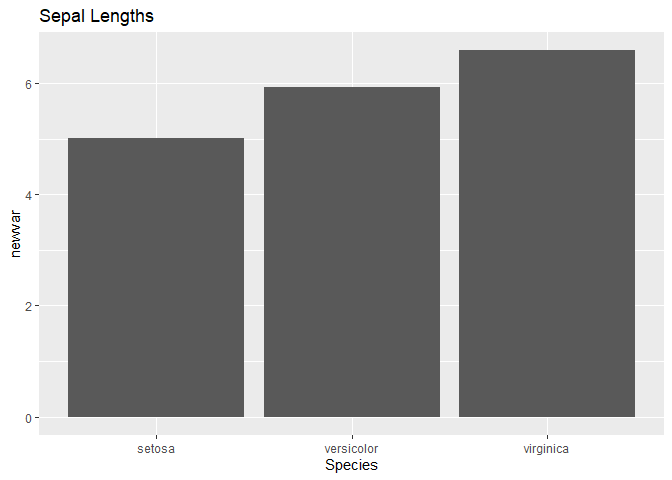# Review: Writing Functions

Before we start developing our very own R Packages, let’s review the basic building blocks of R programming: functions.Time Estimates:Videos: 55 minReadings: 30 minActivities: 0 minCheck-ins: 6Extra Resources:

## Basics of Functions

If you do not recall the basics of writing functions, or if you want a quick refresher, watch the video below.Optional Video: How to Write a Function

### Anatomy of a function definition

Let’s establish some vocabulary moving forward. Consider the very simple function below:

``````add_or_subtract <- function(first_num, second_num = 2, type = "add") {

first_num + second_num
} else if (type == "subtract") {
first_num - second_num
} else {
}

}``````

The function name is chosen by whoever writes the function:

``add_or_subtract <- function(first_num, second_num = 2, type = "add") {    if (type == "add") {    first_num + second_num  } else if (type == "subtract") {    first_num - second_num  } else {    stop("Please choose `add` or `subtract` as the type.")  }  }``

The required arguments are the ones for which no default value is supplied:

``add_or_subtract <- function(first_num, second_num = 2, type = "add") {    if (type == "add") {    first_num + second_num  } else if (type == "subtract") {    first_num - second_num  } else {    stop("Please choose `add` or `subtract` as the type.")  }  }``

The optional arguments are the ones for which a default value is supplied.

``add_or_subtract <- function(first_num, second_num = 2, type = "add") {    if (type == "add") {    first_num + second_num  } else if (type == "subtract") {    first_num - second_num  } else {    stop("Please choose `add` or `subtract` as the type.")  }  }``

The body of the function is all the code inside the definition. This code will be run in the environment of the function, rather than in the global environment. This means that code in the body of the function does not have the power to alter anything outside the function.

(There are ways to cheat your way around this… we will avoid them!)

``add_or_subtract <- function(first_num, second_num = 2, type = "add") {    if (type == "add") {    first_num + second_num  } else if (type == "subtract") {    first_num - second_num  } else {    stop("Please choose `add` or `subtract` as the type.")  }  }``

The return values of the function are the possible objects that get returned:

``add_or_subtract <- function(first_num, second_num = 2, type = "add") {    if (type == "add") {    first_num + second_num  } else if (type == "subtract") {    first_num - second_num  } else {    stop("Please choose `add` or `subtract` as the type.")  }  }``

When we use a function in code, this is referred to as a function call.

``b <- add_or_subtract(1, 2)``Check-In 1: Function Basics

Question 1: What will be returned by each of the following?

1. 1
2. -1
3. 30
4. An error defined in the function `add_or_subtract`
5. An error defined in a different function, that is called from inside `add_or_subtract`
``````add_or_subtract(5, 6, type = "subtract")

Question 2:

Consider the following code:

``````first_num <- 5
second_num <- 3

result <- 8

result <- add_or_subtract(first_num, second_num = 4)

In your Global Environment, what is the value of…

1. `first_num`
2. `second_num`
3. `result`
4. `result_2`Canvas Link

## Good function design

Most likely, you have so far only written functions for your own convenience. (Or for assignments, of course!)

We are now going to be designing functions for other people to use and possibly even edit them. This means we need to put some thought into the design of the function.Required Reading: R4DS Chapter 19: Functions

Designing functions is somewhat subjective, but there are a few principles that apply:

1. Choose a good, descriptive names
• Your function name should describe what it does, and usually involves a verb.
• Your argument names should be simple and/or descriptive.
• Names of variables in the body of the function should be descriptive.
2. Output should be very predictable
• Your function should always return the same object type, no matter what input it gets.
• Your function should expect certain objects or object types as input, and give errors when it does not get them.
• Your function should give errors or warnings for common mistakes.
• Default values of arguments should only be used when there is a clear common choice.
3. The body of the function should be easy to read.
• Code should use good style principles.
• There should be occasional comments to explain the purpose of the steps.
• Complicated steps, or steps that are repeated many times, should be written into separate functions (sometimes called helper functions).
4. Functions should be self-contained.
• They should not rely on any information besides what is given as input.
• (Relying on other functions is fine, though)
• They should not alter the Global Environment
• (do not put `library()` statements inside functions!)Check-In 2: Function Design

Identify five major violations of design principles for the following function:

``````ugh <- function(dataset) {

library(tidyverse)

thing <- dataset%>%group_by(Species)%>%summarize(newvar = mean(Sepal.Length))

ggplot(thing)+geom_col(aes(x = Species, y = newvar))+ggtitle("Sepal Lengths")

}

ugh(iris)``````Canvas Link

## Debugging Functions

Suppose you’ve done it: You’ve written the most glorious, beautiful, well-designed function of all time. It’s many lines long, and it relies on several sub-functions.

You run it and - it doesn’t work.